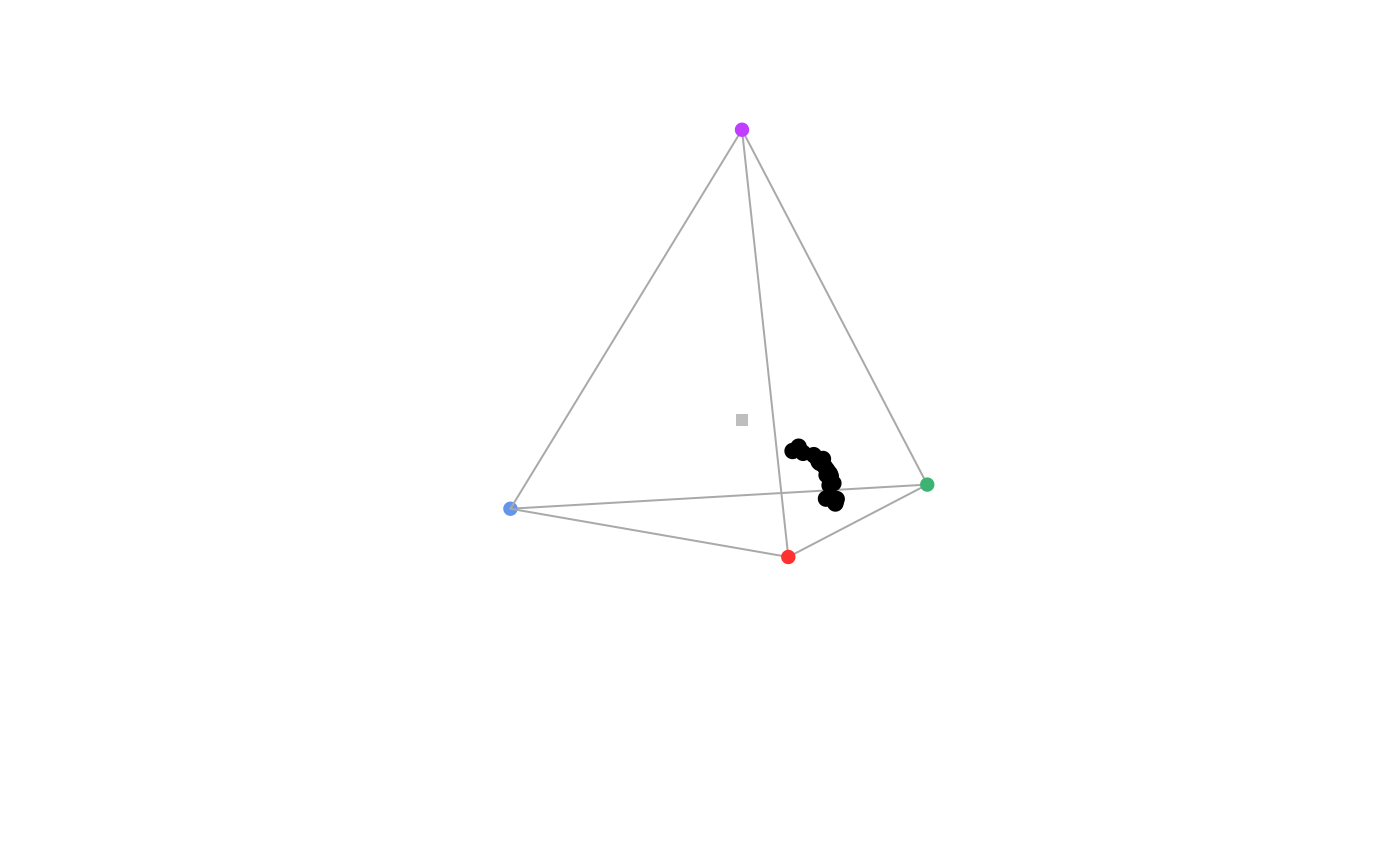Produces a static 3D tetrahedral plot.

tetraplot(
tcsdata,
theta = 45,
phi = 10,
perspective = FALSE,
range = c(1, 2),
r = 1e+06,
zoom = 1,
achro = TRUE,
achro.col = "grey",
achro.size = 1,
achro.line = FALSE,
achro.lwd = 1,
achro.lty = 3,
tetrahedron = TRUE,
vert.cex = 1,
vert.range = c(1, 2),
out.lwd = 1,
out.lcol = "darkgrey",
type = "p",
labels = FALSE,
gamut = FALSE,
margin = NULL,
...
)

## Arguments

tcsdata (required) a data frame, possibly a result from the colspace() or tcspace() function, containing values for the 'x', 'y' and 'z' coordinates as columns (labeled as such). angle to rotate the plot in the xy plane (defaults to 45). angle to rotate the plot in the yz plane (defaults to 10). logical, should perspective be forced by using point size to reflect distance from the plane of view? (defaults to FALSE) vert.range multiplier applied to cex and vert.cex, respectively, to indicate the size range variation reflecting the distance from the plane of view. the distance of the eyepoint from the center of the plotting box. Very high values approximate an orthographic projection (defaults to 1e6). See persp() for details. zooms in (values greater than 1) or out (values between 0 and 1) from the plotting area. should a point be plotted at the origin (defaults to TRUE)? logical. Should the achromatic line be plotted? (defaults to FALSE) graphical parameters for the achromatic coordinates. logical. Should the tetrahedron be plotted? (defaults to TRUE) size of the points at the vertices (defaults to 1). graphical parameters for the tetrahedral outline. accepts a vector of length 1 or 2 with 'p' for points and/or 'l' for lines from the point to the base of the tetrahedron. logical. Should the name of each cone be printed next to the corresponding vertex? logical. Should the polygon showing the possible colours given visual system and illuminant used in the analysis (defaults to FALSE). This option currently only works when qcatch = Qi. Deprecated. Please use the standard par() method for custom margins. additional graphical options. See par().

## Value

tetraplot() creates a non-interactive 3D plot.

Stoddard, M. C., & Prum, R. O. (2008). Evolution of avian plumage color in a tetrahedral color space: A phylogenetic analysis of new world buntings. The American Naturalist, 171(6), 755-776.

Endler, J. A., & Mielke, P. (2005). Comparing entire colour patterns as birds see them. Biological Journal Of The Linnean Society, 86(4), 405-431.

## Author

Rafael Maia rm72@zips.uakron.edu

Thomas White thomas.white026@gmail.com`